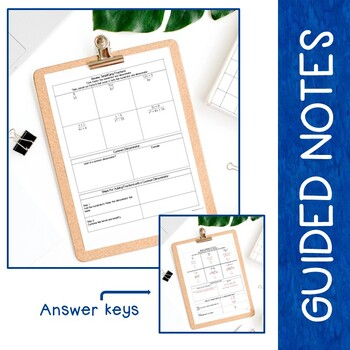# Adding + Subtracting Rational Expressions - Guided Notes, Presentation, + INB

Rated 5 out of 5, based on 17 reviews
17 Ratings;
7th - 10th, Homeschool
Subjects
Resource Type
Standards
Formats Included
• Zip
Pages
28 pages
Report this resource to TPT
##### Also included in
1. This Algebra 1 Bundle is flexible, editable, and can be used for in-person or distance learning. Take the time and stress out of creating your own guided notes and presentations. There are many ways to use this engaging bundle. Students can either build interactive math notebooks with guided notes (
Price \$55.60Original Price \$69.50Save \$13.90
2. This HUGE (over 1,000 pages) high school bundle contains a full-year of guided/scaffolded notes and interactive notebook activities for an entire year of Algebra 1, Geometry, and Algebra 2. The Algebra 1 bundle contains 11 units of study plus 2 mini-units, the Geometry bundle contains 13 units, and
Price \$180.74Original Price \$258.20Save \$77.46
3. This HUGE (over 500 pages) Algebra curriculum pack contains three of our full-year, best selling bundles: Guided Notes Bundle (scaffolded notes, presentation/presentation handouts, INB activities, quick checks for understanding), Student Practice Pages Bundle, and Algebra 1 Assessments Bundle. All
Price \$90.72Original Price \$129.60Save \$38.88
4. This product contains three full years of editable content (Algebra 1, Geometry, Algebra 2) for the high school mathematics classroom. It includes Guided Notes Bundles (guided notes, presentation, presentation handouts, INB foldable activities, and quick checks for understanding), Student Practice P
Price \$320.15Original Price \$492.55Save \$172.40

### Description

This flexible resource on Adding and Subtracting Rational Expressions allows students to either build interactive math notebooks with guided notes (keys included) and foldable activities OR use the included presentation handouts (keys included) with the PowerPoint presentation for focused instruction. Quick checks for understanding (keys included) help to determine how well your students understand the material as you go. Choose what works best for your class and modify to make the content fit your needs. Notes are designed to clearly present the topics and reach all types of learners.

The zip file includes BOTH editable, plain-font resources and ready-to-print, non-editable PDFs. Suggested use guides are included.

Interactive Math Notebook FORMAT – Includes 3 parts: guided notes, foldable activities, and quick checks for understanding. Piece the components together and students can create their own “math textbook” in a math journal.

Presentation Sheets FORMAT – Include 2 parts: guided notes and quick checks for understanding. They are organized in the same order as the included PowerPoint and are designed to print and present. The quick checks can be cut off the bottom of the note sheets and turned in as an assessment tool.

Presentation – Plain-font, no frills, PowerPoint presentation that you can use to present content to students. Slides have blank, student note templates followed by filled in teacher keys. Modify the editable presentation to best fit your needs.

Topics presented in this product include:

• Review: Simplifying Fractions
• What is a Common Denominator?
• Steps for Adding Fractions with a Common Denominator
• Adding Fractions with a Common Denominator
• Steps for Subtracting Fractions with a Common Denominator
• Distribute Before Subtracting Fractions
• Adding/Subtracting Fractions with Different Denominators
• Adding/Subtracting an Integer and a Fraction
• Adding/Subtracting a Polynomial and a Fraction
• Finding the Least Common Denominator (Denominators are Integers)
• Finding the Least Common Denominator (Denominators are Terms)
• Finding the Least Common Denominator (Denominators are Polynomials)
• Use Common Denominators to Solve Equations
• What is a Proportion?

Adding and Subtracting Rational Expressions Student Practice Pages: One full page of practice for each topic covered in the quick checks for understanding. Includes student pages and teacher keys. Perfect for homework, classwork, or a review.

Adding and Subtracting Rational Expressions Editable Assessments Two editable assessments that pair perfectly with the unit.

Related Products:

**Full-year Curriculum Packs**

Algebra 1, Geometry, Algebra 2 Curriculum Pack MEGA BUNDLE

Algebra 1 Curriculum Pack BUNDLE

Geometry Curriculum Pack BUNDLE

Algebra 2 Curriculum Pack BUNDLE

Scaffolded Notes/Interactive Notebook Bundles

Algebra 1 INB Bundle

Geometry INB Bundle

Algebra 2 INB Bundle

Pre-Calculus INB Bundle

Pre-Calculus Add on for Algebra 2 INB Bundle

Statistics INB Bundle

High School Math Interactive Notebook Bundle (Algebra 1, Geometry, Algebra 2)

Student Practice Pages Bundles

Algebra 1 Student Practice Pages Bundle

Geometry Student Practice Pages Bundle

Algebra 2 Student Practice Pages Bundle

High School Math Student Practice Pages Bundle (Algebra 1, Geometry, Algebra 2)

Assessment Bundles

Algebra 1 Assessment Bundle

Geometry Assessment Bundle

Algebra 2 Assessment Bundle

High School Math Assessment Bundle (Algebra 1, Geometry, Algebra 2)

Consumer Math

Consumer Math Bundle

**If you like what you see, please click on the "Follow Me" star to learn about new products, sales, and more!

Total Pages
28 pages
N/A
Teaching Duration
N/A
Report this resource to TPT
Reported resources will be reviewed by our team. Report this resource to let us know if this resource violates TPT’s content guidelines.

### Standards

to see state-specific standards (only available in the US).
Use the structure of an expression to identify ways to rewrite it. For example, see 𝘹⁴ – 𝘺⁴ as (𝘹²)² – (𝘺²)², thus recognizing it as a difference of squares that can be factored as (𝘹² – 𝘺²)(𝘹² + 𝘺²).
Choose and produce an equivalent form of an expression to reveal and explain properties of the quantity represented by the expression.
Understand that polynomials form a system analogous to the integers, namely, they are closed under the operations of addition, subtraction, and multiplication; add, subtract, and multiply polynomials.
Rewrite simple rational expressions in different forms; write 𝘢(𝘹)/𝘣(𝘹) in the form 𝘲(𝘹) + 𝘳(𝘹)/𝘣(𝘹), where 𝘢(𝘹), 𝘣(𝘹), 𝘲(𝘹), and 𝘳(𝘹) are polynomials with the degree of 𝘳(𝘹) less than the degree of 𝘣(𝘹), using inspection, long division, or, for the more complicated examples, a computer algebra system.
Understand that rational expressions form a system analogous to the rational numbers, closed under addition, subtraction, multiplication, and division by a nonzero rational expression; add, subtract, multiply, and divide rational expressions.